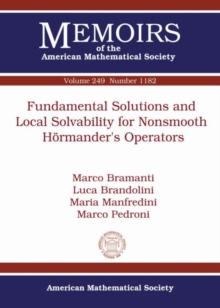Supporting your high street Find out how »
• My Account# Fundamental Solutions and Local Solvability for Nonsmooth Hormander's Operators Paperback / softback

## Part of the Memoirs of the American Mathematical Society series

#### Description

The authors consider operators of the form $L=\sum_{i=1}^{n}X_{i}^{2}+X_{0}$ in a bounded domain of $\mathbb{R}^{p}$ where $X_{0},X_{1},\ldots,X_{n}$ are nonsmooth Hormander's vector fields of step $r$ such that the highest order commutators are only Holder continuous.

Applying Levi's parametrix method the authors construct a local fundamental solution $\gamma$ for $L$ and provide growth estimates for $\gamma$ and its first derivatives with respect to the vector fields.

Requiring the existence of one more derivative of the coefficients the authors prove that $\gamma$ also possesses second derivatives, and they deduce the local solvability of $L$, constructing, by means of $\gamma$, a solution to $Lu=f$ with Holder continuous $f$.

The authors also prove $C_{X,loc}^{2,\alpha}$ estimates on this solution.

#### Information

• Format: Paperback / softback
• Pages: 79 pages
• Publisher: American Mathematical Society
• Publication Date:
• Category: Algebra
• ISBN: 9781470425593

£71.95

£71.55

on all orders

###### Pick up orders

from local bookshops

£49.99

£40.79

£49.99

£42.49

£99.99

£98.55

£99.99

£81.45

£77.50

£76.95

£77.50

£76.95

£77.50

£76.95

£77.50

£76.95